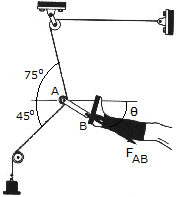# Engineering Mechanics - Equilibrium of a Particle - Discussion

### Discussion :: Equilibrium of a Particle - General Questions (Q.No.4)

4.Determine the magnitude and directionof the resultant force FAB exerted along link AB by the tractive apparatus shown. The suspended mass is 10 kg. Neglect the size of the pulley at A.

 [A]. FAB = 170.0 N,= 75.0° [B]. FAB = 170.0 N,= 15.0° [C]. FAB = 98.1 N,= 75.0° [D]. FAB = 98.1 N,= 15.0°

Explanation:

No answer description available for this question.

 Pratik/Satish India said: (Aug 28, 2011) Applying Lami's theorem , angle between horizontal plane and Link AB is 15 degree and again by lami's theorem Reaction AB is 10Kg i.e. 98.1 N. 10/Sin(105+θ)= 10/sin(135-θ) so θ= 15 Now 10/Sin(105+θ) = Fab/sin(120) So Fab = 10 Kg = 9.81*10 Kg = 98.1 N.

 P.Velmurugan said: (Sep 30, 2011) Five forces of 100,200,300,400,and 500Nare acting at angles of 40deg,100 deg,210deg,280 deg,and 340 degin anticlockwise direction from the X-axis at a point ,all acting away from the point. Find the resultant force in magnitude and direction.

 P.Velmurugan said: (Sep 30, 2011) Determine analytically the resultant of the coplanar forces acting vertically- upwards.1)40N 2)20N at 30CM 3)30N at 50CM 4)60N at 70 CM.

 P.Velmurugan said: (Sep 30, 2011) Resolve a force of 200N into two components making angles of 60deg and 45deg with it on opposite sides.

 Prince Tetteh said: (Mar 13, 2015) The weight of the object is experienced throughout the string in the pulley. W = mg = 10*9.81 = 98.1 N. Summation of forces along x-axis = 0. F(AB) cos\$-98.1 cos45-98.1 cos75 = 0. F(AB) cos\$ = 94.757 N ------- Equation 1. Summation of forces along y-axis = 0. F(AB) sin\$-98.1sin75+98.1sin45 = 0. F(AB) sin\$ = 25.39 N -------- Equation 2. Equation 2/Equation 1. tan\$ = 0.2679 \$ = 15 deg. From Equation 1. F(AB) = 94.757/cos15 = 98.09 N. Therefore D.